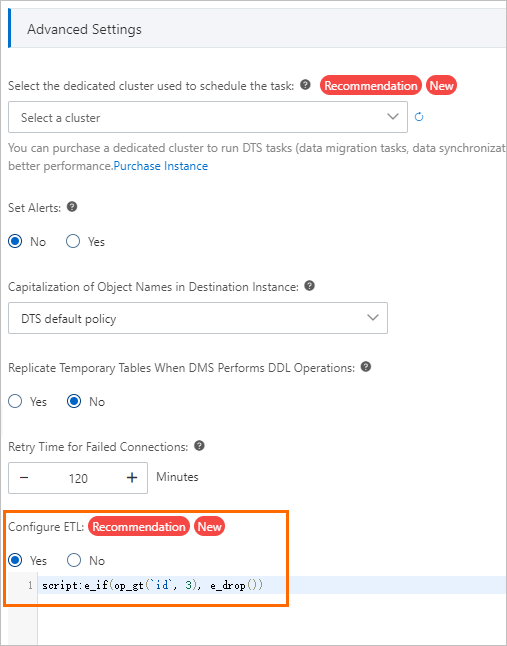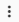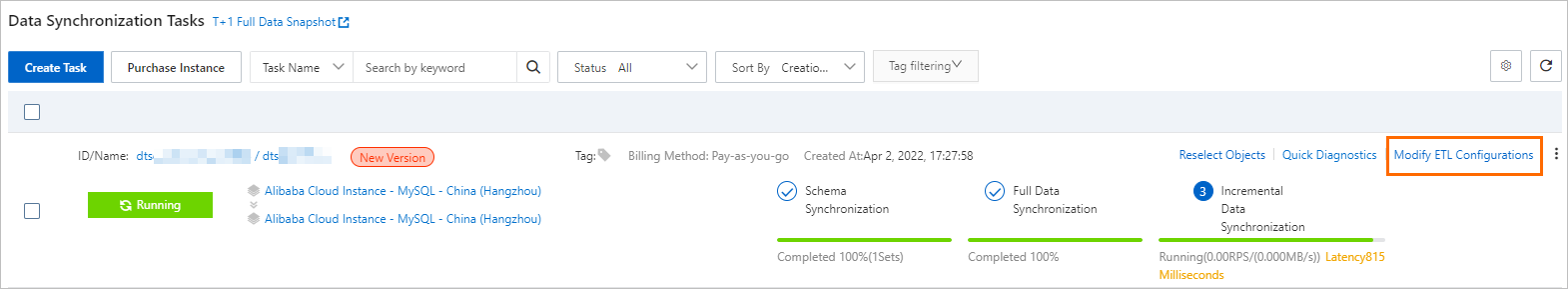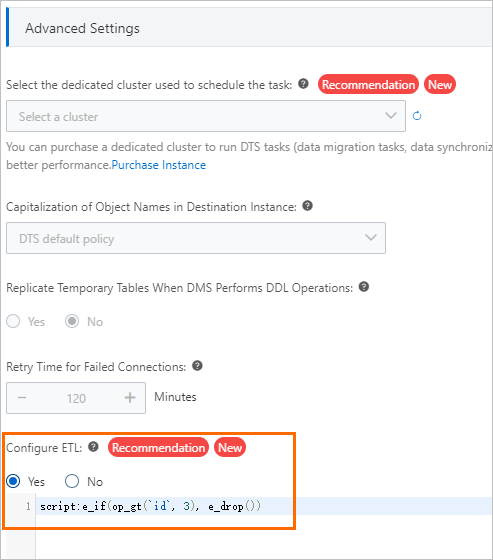Data Transmission Service (DTS) provides the extract, transform, and load (ETL) feature to help you process streaming data in real time. For more information, see What is ETL? The ETL feature is integrated with the data replication capabilities of DTS to achieve streaming data extraction, data transformation and processing, and data loading. The ETL feature can be used in the following scenarios: data filtering, data masking, recording of data modification time, and data change audit. This topic describes how to configure ETL in a data synchronization task.

## Background information

DTS is used for data migration and real-time data transmission between data sources. In some cases, you may need to transform or filter real-time data before the data is written to a database. To meet such requirements, DTS provides the ETL feature and allows you to use domain-specific language (DSL) statements to process data in a flexible manner. For more information about DSL, see Overview of DSL.

You can configure an ETL task by using one of the following methods:
Note Both data migration and data synchronization tasks allow you to configure ETL. This topic describes how to configure ETL in a data synchronization task. You can also follow the procedure to configure ETL in a data migration task.

## Supported databases

The following table lists the source and destination databases that are supported by the ETL feature.

Source database Destination database
SQL Server
• AnalyticDB for MySQL V3.0
• SQL Server
• MySQL
• PolarDB for MySQL
MySQL
• AnalyticDB for MySQL V3.0
• AnalyticDB for PostgreSQL
• Kafka
• MySQL
• PolarDB for MySQL
Self-managed Oracle database
• AnalyticDB for MySQL V3.0
• AnalyticDB for PostgreSQL
• Kafka
• MaxCompute
• PolarDB-X 2.0
• PolarDB for Oracle
PolarDB for MySQL
• AnalyticDB for MySQL V3.0
• MySQL
• PolarDB for MySQL
PolarDB for Oracle
• AnalyticDB for MySQL V3.0
• PolarDB for Oracle
PolarDB-X 1.0
• Kafka
• Tablestore
Self-managed Db2 for LUW database MySQL
Self-managed Db2 for i database MySQL
PolarDB for PostgreSQL
• PolarDB for PostgreSQL
• PostgreSQL
PostgreSQL
• PolarDB for PostgreSQL
• PostgreSQL
TiDB
• PolarDB for MySQL
• MySQL

## Configure ETL when you create a data synchronization task

Usage notes

If the ETL script that you configure contains the operation to add a column, you must manually add a column to the destination table. Otherwise, the ETL script does not take effect. For example, if you configure the ETL script `script:e_set(`new_column`, dt_now())`, you must manually add the `new_column` column to the destination table.

2. In the Advanced Settings section of the Configure Objects and Advanced Settings step, set the Configure ETL parameter to Yes. In the code editor, enter data processing statements in accordance with the DSL syntax.
Note If you want to drop entries whose values of the id column are greater than 3 by using DSL, you can use the `script:e_if(op_gt(`id`, 3), e_drop())` statement. In this statement, `op_gt` is an expression function used to determine whether an entry is greater than a specific value. `id` is a variable. This way, entries whose values of the id column are greater than 3 are filtered out.3. Click Next: Save Task Settings and Precheck and complete the subsequent steps.

## Modify the ETL configurations of an existing data synchronization task

You can modify the ETL configurations in the following scenarios:
• If the Configure ETL parameter is set to No for an existing data synchronization task, you can set this parameter to Yes and write the DSL script.
• If the Configure ETL parameter is set to Yes for an existing data synchronization task, you can modify the existing DSL script or set this parameter to No.
Usage notes
• For an existing data synchronization task, you cannot make changes to the destination table schema by modifying the ETL configurations of the task. You can modify the destination table schema only before the data synchronization task is started.
• If you modify the ETL configurations, the data synchronization task may be interrupted. Proceed with caution.
• The modification of ETL configurations takes effect only on the incremental data generated after the modification.
1. Find the data synchronization task for which you want to modify ETL configurations and click Modify ETL Configurations next to theicon.2. In the Advanced Settings section of the Configure Objects and Advanced Settings step, set the Configure ETL parameter to Yes. In the code editor, enter data processing statements in accordance with the DSL syntax.
Note If you want to drop entries whose values of the id column are greater than 3 by using DSL, you can use the `script:e_if(op_gt(`id`, 3), e_drop())` statement. In this statement, `op_gt` is an expression function used to determine whether an entry is greater than a specific value. `id` is a variable. This way, entries whose values of the id column are greater than 3 are filtered out.3. Click Next: Precheck and Start Task and complete the subsequent steps.

## Overview of DSL

DSL is a scripting language that is designed to process data in data synchronization scenarios. You can use conditional functions to process data of the string, date, and numeric types. DSL boasts the following characteristics that help you process data in a flexible manner:
• Various functions: DSL provides a variety of functions and supports combined functions.
• Simple syntax: DSL is easy to use. For more information about how to use DSL to filter, convert, and mask data, see Typical scenarios.
• High efficiency: DSL has a minimal effect on the data synchronization performance because DSL uses the code generation mechanism.
Note DSL syntax has something in common with the DSL for Log Service. For more information about the DSL for Log Service, see Syntax overview.

## Typical scenarios

• Filter data
• Filter out data by a numeric column: If an entry whose value of the id column is greater than 10000, drop this entry so that it is not synchronized to the destination database. Example: e_if(op_gt(`id`, 10000), e_drop).
• Filter out data by a specific string: If an entry whose value of the name column contains "hangzhou", drop this entry so that it is not synchronized to the destination database. Example: e_if(str_contains(`name`, "hangzhou"), e_drop).
• Filter out data by date: If an entry whose timestamp of the order column is earlier than a specific point in time, drop this entry so that it is not synchronized to the destination database. Example: e_if(op_lt(`order_timestamp`, "2015-02-23 23:54:55"), e_drop).
• Filter out data by multiple conditions:
• If an entry whose value of the id column is greater than 1000 and value of the name column contains "hangzhou", drop this entry so that it is not synchronized to the destination database. Example: e_if(op_and(str_contains(`name`, "hangzhou"), op_gt(`id`, 1000)), e_drop()).
• If an entry whose value of the id column is greater than 1000 or value of the name column contains "hangzhou", drop this entry so that it is not synchronized to the destination database. Example: e_if(op_or(str_contains(`name`, "hangzhou"), op_gt(`id`, 1000)), e_drop()).
• Mask the last four digits of a mobile phone number with four asterisks (*). Example: e_set(`phone`, str_mask(`phone`, 7, 10, '*')).
• Record the time when data is modified
• Add a column to all tables: If the value of the __OPERATION__ variable is INSERT, UPDATE, or DELETE, a column named dts_sync_time whose value is the same as the __COMMIT_TIMESTAMP__ variable of logs is added to all tables in the source database.
``````e_if(op_or(op_or(
op_eq(__OPERATION__, __OP_INSERT__),
op_eq(__OPERATION__, __OP_UPDATE__)),
op_eq(__OPERATION__, __OP_DELETE__)),
e_set(dts_sync_time, __COMMIT_TIMESTAMP__))``````
• Add a column to a specific table: If the value of the __OPERATION__ variable is INSERT, UPDATE, or DELETE, a column named dts_sync_time whose value is the same as the __COMMIT_TIMESTAMP__ variable of logs is added to the dts_test_table table in the source database.
``````e_if(op_and(
op_eq(__TB__,'dts_test_table'),
op_or(op_or(
op_eq(__OPERATION__,__OP_INSERT__),
op_eq(__OPERATION__,__OP_UPDATE__)),
op_eq(__OPERATION__,__OP_DELETE__))),
e_set(dts_sync_time,__COMMIT_TIMESTAMP__))``````
Note To perform the preceding operations, you must add the dts_sync_time column to the corresponding tables in the destination database before the data synchronization task is started.
• Audit data changes
• Record the type and time of the data changes in tables: 1. Record the data change type in the operation_type column of the destination database. 2. Record the time when data is changed in the updated column of the destination database.
``````e_compose(
e_switch(
op_eq(__OPERATION__,__OP_DELETE__), e_set(operation_type, 'DELETE'),
op_eq(__OPERATION__,__OP_UPDATE__), e_set(operation_type, 'UPDATE'),
op_eq(__OPERATION__,__OP_INSERT__), e_set(operation_type, 'INSERT')),
e_set(updated, __COMMIT_TIMESTAMP__),
e_set(__OPERATION__,__OP_INSERT__)
)``````
Note You must add the operation_type and updated columns to the tables in the destination database before the data synchronization task is started.

## DSL syntax

Constants and variables
• Constants
Data type Example
INT 123
FLOAT 123.4
STRING "hello1_world"
BOOLEAN true or false
DATETIME '2021-01-01 10:10:01'
• Variables
Variable Description Data type Example
__TB__ The name of the table. STRING table
__DB__ The name of the database. STRING mydb
__OPERATION__ The type of the operation. STRING __OP_INSERT__,__OP_UPDATE__,__OP_DELETE__
__COMMIT_TIMESTAMP__ The time when the transaction was committed. DATETIME '2021-01-01 10:10:01'
`column` The name of the column. STRING `id` or `name`
Expression functions
• Arithmetic operators
Operation Syntax Valid value Return value Example
• value1: an integer or a floating-point number
• value2: an integer or a floating-point number
If value1 and value2 are integers, an integer is returned. Otherwise, a floating-point number is returned. op_sum(`col1`, 1.0)
Subtraction op_sub(value1, value2)
• value1: an integer or a floating-point number
• value2: an integer or a floating-point number
If value1 and value2 are integers, an integer is returned. Otherwise, a floating-point number is returned. op_sub(`col1`, 1.0)
Multiplication op_mul(value1, value2)
• value1: an integer or a floating-point number
• value2: an integer or a floating-point number
If value1 and value2 are integers, an integer is returned. Otherwise, a floating-point number is returned. op_mul(`col1`, 1.0)
Division op_div_true(value1, value2)
• value1: an integer or a floating-point number
• value2: an integer or a floating-point number
If value1 and value2 are integers, an integer is returned. Otherwise, a floating-point number is returned. op_div_true(`col1`, 2.0). In this example, if the col1 value is 15, 7.5 is returned.
Modulo op_mod(value1, value2)
• value1: an integer or a floating-point number
• value2: an integer or a floating-point number
If value1 and value2 are integers, an integer is returned. Otherwise, a floating-point number is returned. op_mod(`col1`, 10). In this example, if the col1 value is 23, 3 is returned.
• Logical operators
Operation Syntax Valid value Return value Example
Equal to op_eq(value1, value2)
• value1: an integer, a floating-point number, or a string
• value2: an integer, a floating-point number, or a string
true or false op_eq(`col1`, 23)
Greater than op_gt(value1, value2)
• value1: an integer, a floating-point number, or a string
• value2: an integer, a floating-point number, or a string
true or false op_gt(`col1`, 1.0)
Less than op_lt(value1, value2)
• value1: an integer, a floating-point number, or a string
• value2: an integer, a floating-point number, or a string
true or false op_lt(`col1`, 1.0)
Greater than or equal to op_ge(value1, value2)
• value1: an integer, a floating-point number, or a string
• value2: an integer, a floating-point number, or a string
true or false op_ge(`col1`, 1.0)
Less than or equal to op_le(value1, value2)
• value1: an integer, a floating-point number, or a string
• value2: an integer, a floating-point number, or a string
true or false op_le(`col1`, 1.0)
AND op_and(value1, value2)
• value1: a Boolean value
• value2: a Boolean value
true or false op_and(`is_male`, `is_student`)
OR op_or(value1, value2)
• value1: a Boolean value
• value2: a Boolean value
true or false op_or(`is_male`, `is_student`)
• String functions
Purpose Syntax Valid value Return value Example
Format strings and append strings str_format(format, value1, value2, value3, ...)
• format: a string. Braces ({}) are used as placeholders. Example: "part1: {}, part2: {}".
• value1: an arbitrary value.
• value2: an arbitrary value.
The string after the format operation. str_format("part1: {}, part2: {}", `col1`, `col2`). In this example, if the col1 value is ab and the col2 value is 12, "part1: ab, part2: 12" is returned.
Replace strings str_replace(original, oldStr, newStr, count)
• original: the original string.
• oldStr: the string to be replaced.
• newStr: the string after the replacement.
• count: an integer that indicates the maximum number of times that a string can be replaced. A value of -1 indicates that all oldStr is replaced with newStr.
The string after the replace operation. Example 1: str_replace(`name`, "a", 'b', 1). In this example, if the name is aba, bba is returned. Example 2: str_replace(`name`, "a", 'b', -1). In this example, if the name is aba, bbb is returned.
Remove specific characters at the start and end of a string str_strip(string_val, charSet)
• string_val: the original string.
• char_set: the set of the first characters and the last characters of the string.
The string after the remove operation. str_strip(`name`, 'ab'). In this example, if the name is axbzb, xbz is returned.
Convert strings to lowercase letters str_lower(value1) value1: a column of the string type or a string constant. The string after the convert operation. str_lower(`str_col`)
Convert strings to uppercase letters str_upper(value1) value1: a column of the string type or a string constant. The string after the convert operation. str_upper(`str_col`)
Count strings str_count(str, pattern)
• str: a column of the string type or a string constant.
• pattern: the substring to query.
The number of times for which the substring appears. str_count(`str_col`, 'abc'). In this example, if the str_col value is zabcyabcz, 2 is returned.
Query strings str_find(str, pattern)
• str: a column of the string type or a string constant.
• pattern: the substring to query.
The position in which the substring matches for the first time. If no match is found, -1 is returned. str_find(`str_col`, 'abc'). In this example, if the str_col value is xabcy, 1 is returned.
Determine whether a string contains only letters str_isalpha(str) str: a column of the string type or a string constant. true or false str_isalpha(`str_col`)
Determine whether a string contains only digits str_isdigit(str) str: a column of the string type or a string constant. true or false str_isdigit(`str_col`)
Mask part of a string with specific characters. This operation can be used for data masking. For example, mask the last four digits of a mobile phone number with four asterisks (*). str_mask(str, start, end, maskStr)
• str: a column of the string type or a string constant.
• start: an integer that indicates the start position of the masking. The minimum value is 0.
• end: an integer that indicates the end position of the masking. The maximum value is the length of the string minus 1.
• maskStr: a string. The length is 1. Example: #.
The string whose part from start to end is masked with specified characters. str_mask(`phone`, 7, 10, '#')
Query the DateTime value of the current time zone dt_now() None The DateTime value of the current time zone. e_set(`dt_col`, dt_now())
Query the DateTime value of the current time zone in UTC dt_utcnow() None The DateTime value of the current time zone in UTC. e_set(`dt_col`, dt_utcnow())
Global functions
• Flow control functions
Purpose Syntax Description Example
The IF statement e_if(bool_expr, func_invoke)
• bool_expr: a Boolean constant or a function. A Boolean constant: true or false. A function: op_gt(`id`, 10).
• func_invoke: a function. Valid values: e_drop, e_keep, e_set, e_if, and e_compose.
e_if(op_gt(`id`, 10), e_drop()). In this example, if an entry whose value of the id column is greater than 10, this entry is dropped.
The IF ELSE statement e_if_else(bool_expr, func_invoke1, func_invoke2)
• bool_expr: a Boolean constant or a function. A Boolean constant: true or false. A function: op_gt(`id`, 10).
• func_invoke1: a function. Invoke this function if the condition is true.
• func_invoke2: a function. Invoke this function if the condition is false.
e_if_else(op_gt(`id`, 10), e_set(`tag`, 'large'), e_set(`tag`, 'small')). In this example, if an entry whose value of the id column is greater than 10, the tag column is set to large. Otherwise, the tag column is set to small.
The SWITCH statement that contains multiple conditions and a default operation s_switch(condition1, func1, condition2, func2, ..., default = default_func)
• condition1: a Boolean constant or a function. A Boolean constant: true or false. A function: op_gt(`id`, 10).
• func_invoke: a function. If condition1 is true, invoke this function and finish the statement. Otherwise, proceed to the next condition.
• default_func: a function. If the preceding conditions are false, invoke this function.
e_switch(op_gt(`id`, 100), e_set(`str_col`, '>100'), op_gt(`id`, 90), e_set(`str_col`, '>90'), default=e_set(`str_col`, '<=90'))
Combine multiple operations e_compose(func1, func2, func3, ...)
• func1: a function. Valid values: e_set, e_drop, and e_if.
• func2: a function. Valid values: e_set, e_drop, and e_if.
e_compose(e_set(`str_col`, 'test'), e_set(`dt_col`, dt_now())). In this example, the value of the str_col column is set to test, and the value of the dt_col column is set to the current time.
• Data manipulation functions
Purpose Syntax Description Example
Drop this entry so that it is not synchronized to the destination database e_drop() None e_if(op_gt(`id`, 10), e_drop()). In this example, entries whose values of the id column are greater than 10 are dropped.
Retain this entry so that it is synchronized to the destination database e_keep(condition) condition: a Boolean expression. e_keep(op_gt(id, 1)). In this example, only entries whose values of the id column are greater than 1 are synchronized.
Set the value of a column e_set(`col`, val)
• col: the name of the column.
• val: a constant or a function. The data type of the val value must match the data type of the col value.
• e_set(`dt_col`, dt_now()). In this example, the value of the dt_col column is set to the current time.
• e_set(`col1`, `col2` + 1). In this example, the value of the col1 column is set to the value of the col2 column plus 1.